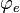# User:IssaRice/Computability and logic/Index and program

In computability theory, if we have some numbering$\varphi_0, \varphi_1, \varphi_2, \ldots$ of computable partial functions then the number$e$ is called the index of$\varphi_e$, i.e.,$\varphi_e$ is the$e$th computable partial function.
We can also think of$e$ as the source code of an algorithm computing$\varphi_e$.